## Einstein's Special Relativity

 - Background - Einstein's Book      (I didn't do well. Ken Shirriff kindly helped my rusty algebra.) - Coursera      (Matches my style :-)) - Relativistic Mass - gravitational vs inertial - E=mc^2 ----------------------- and if you are still not confused, Why No One Has Measured The Speed Of Light from Youtube

### Relativity: The Special and General Theory

by Albert Einstein

A note from this presenter -
 "In the beginning", there was an authorized translation published in London in 1920. Seemingly, all the copies available on the Internet, including this .pdf of the one I purchased via Amazon, were scanned (from that book?), OCRed, (the OCR output corrected to some extent), and converted to HTML and images, before being placed on the Internet. It would be good to compare these/this version with a scan of the actual book, and correct any differences. Assuming the original translation and publication are accurate ;-)) A note for the student (me). In Section 11, "The Lorentz Transformation", Einstein presents the equations of the Lorentz Transformation as a fact, with no derivation - as though handed down from "above". The reader is left with the task of trying to understand equations such as this.In the note at the end of the Section 11, Einstein anounces that a simple derivation is in Appendix I I now sympathize more with students who say that physical sciences are HARD :-(( Try chewing on some of these: https://en.wikipedia.org/wiki/Lorentz_transformation https://en.wikipedia.org/wiki/Derivations_of_the_Lorentz_transformations https://en.wikipedia.org/wiki/Derivations_of_the_Lorentz_transformations#Einstein.27s_popular_derivation -Ed Thelen, September 20, 2017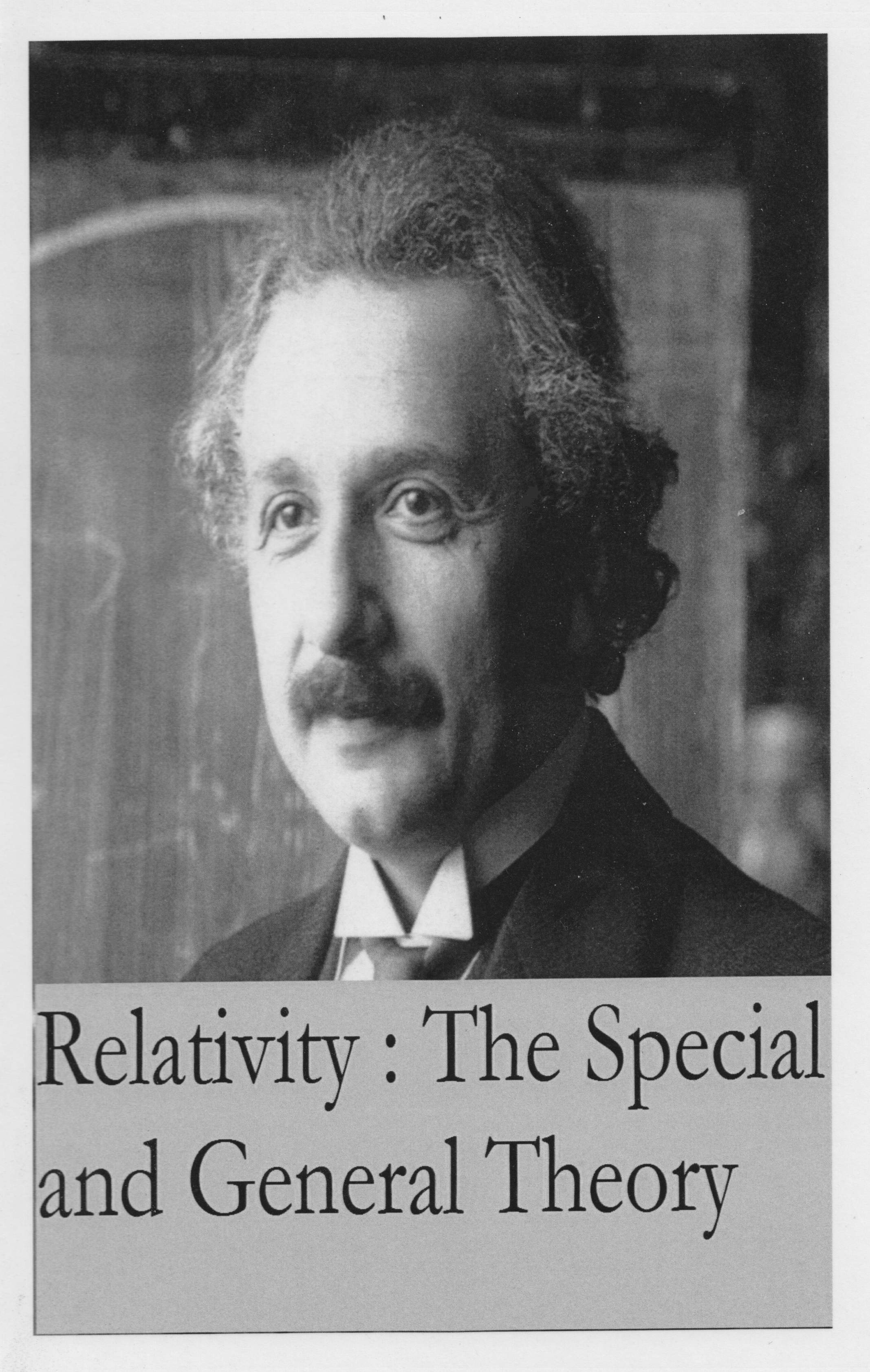``` Title: Relativity: The Special and General Theory Author: Albert Einstein Release Date: February, 2004 [EBook #5001] Edition: 10 Language: English Character set encoding: ASCII ALBERT EINSTEIN REFERENCE ARCHIVE RELATIVITY: THE SPECIAL AND GENERAL THEORY BY ALBERT EINSTEIN Written: 1916 (this revised edition: 1924) Source: Relativity: The Special and General Theory (1920) Publisher: Methuen & Co Ltd First Published: December, 1916 Translated: Robert W. Lawson (Authorised translation) Transcription/Markup: Brian Basgen Transcription to text: Gregory B. Newby Thanks to: Einstein Reference Archive (marxists.org) The Einstein Reference Archive is online at: http://www.marxists.org/reference/archive/einstein/index.htm Transcriber note: This file is a plain text rendition of HTML. Because many equations cannot be presented effectively in plain text, images are supplied for many equations and for all figures and tables. ```

CONTENTS
 Part I: The Special Theory of Relativity Part II: The General Theory of Relativity Part III: Considerations on the Universe as a Whole Appendices: Simple Derivation of the Lorentz Transformation (sup. ch. 11) Minkowski's Four-Dimensional Space ("World") (sup. ch 17) The Experimental Confirmation of the General Theory of Relativity The Structure of Space According to the General Theory of Relativity (sup. ch 32) Relativity and the Problem of Space Note: The fifth Appendix was added by Einstein at the time of the fifteenth re-printing of this book; and as a result is still under copyright restrictions so cannot be added without the permission of the publisher.

PREFACE
 The present book is intended, as far as possible, to give an exact insight into the theory of Relativity to those readers who, from a general scientific and philosophical point of view, are interested in the theory, but who are not conversant with the mathematical apparatus of theoretical physics. The work presumes a standard of education corresponding to that of a university matriculation examination, and, despite the shortness of the book, a fair amount of patience and force of will on the part of the reader. The author has spared himself no pains in his endeavour to present the main ideas in the simplest and most intelligible form, and on the whole, in the sequence and connection in which they actually originated. In the interest of clearness, it appeared to me inevitable that I should repeat myself frequently, without paying the slightest attention to the elegance of the presentation. I adhered scrupulously to the precept of that brilliant theoretical physicist L. Boltzmann, according to whom matters of elegance ought to be left to the tailor and to the cobbler. I make no pretence of having withheld from the reader difficulties which are inherent to the subject. On the other hand, I have purposely treated the empirical physical foundations of the theory in a "step-motherly" fashion, so that readers unfamiliar with physics may not feel like the wanderer who was unable to see the forest for the trees. May the book bring some one a few happy hours of suggestive thought! December, 1916 A. EINSTEIN

PART I : THE SPECIAL THEORY OF RELATIVITY

1. PHYSICAL MEANING OF GEOMETRICAL PROPOSITIONS

2. THE SYSTEM OF CO-ORDINATES
 On the basis of the physical interpretation of distance which has been indicated, we are also in a position to establish the distance between two points on a rigid body by means of measurements. For this purpose we require a " distance " (rod S) which is to be used once and for all, and which we employ as a standard measure. If, now, A and B are two points on a rigid body, we can construct the line joining them according to the rules of geometry ; then, starting from A, we can mark off the distance S time after time until we reach B. The number of these operations required is the numerical measure of the distance AB. This is the basis of all measurement of length.2) Every description of the scene of an event or of the position of an object in space is based on the specification of the point on a rigid body (body of reference) with which that event or object coincides. This applies not only to scientific description, but also to everyday life. If I analyse the place specification " Times Square, New York," 3) I arrive at the following result. The earth is the rigid body to which the specification of place refers; "Times Square, New York," is a well-defined point, to which a name has been assigned, and with which the event coincides in space.4) This primitive method of place specification deals only with places on the surface of rigid bodies, and is dependent on the existence of points on this surface which are distinguishable from each other. But we can free ourselves from both of these limitations without altering the nature of our specification of position. If, for instance, a cloud is hovering over Times Square, then we can determine its position relative to the surface of the earth by erecting a pole perpendicularly on the Square, so that it reaches the cloud. The length of the pole measured with the standard measuring-rod, combined with the specification of the position of the foot of the pole, supplies us with a complete place specification. On the basis of this illustration, we are able to see the manner in which a refinement of the conception of position has been developed. (a) We imagine the rigid body, to which the place specification is referred, supplemented in such a manner that the object whose position we require is reached by. the completed rigid body. (b) In locating the position of the object, we make use of a number (here the length of the pole measured with the measuring-rod) instead of designated points of reference. (c) We speak of the height of the cloud even when the pole which reaches the cloud has not been erected. By means of optical observations of the cloud from different positions on the ground, and taking into account the properties of the propagation of light, we determine the length of the pole we should have required in order to reach the cloud. From this consideration we see that it will be advantageous if, in the description of position, it should be possible by means of numerical measures to make ourselves independent of the existence of marked positions (possessing names) on the rigid body of reference. In the physics of measurement this is attained by the application of the Cartesian system of co-ordinates. This consists of three plane surfaces perpendicular to each other and rigidly attached to a rigid body. Referred to a system of co-ordinates, the scene of any event will be determined (for the main part) by the specification of the lengths of the three perpendiculars or co-ordinates (x, y, z) which can be dropped from the scene of the event to those three plane surfaces. The lengths of these three perpendiculars can be determined by a series of manipulations with rigid measuring-rods performed according to the rules and methods laid down by Euclidean geometry. In practice, the rigid surfaces which constitute the system of co-ordinates are generally not available ; furthermore, the magnitudes? of the co-ordinates are not actually determined by constructions with rigid rods, but by indirect means. If the results of physics and astronomy are to maintain their clearness, the physical meaning of specifications of position must always be sought in accordance with the above considerations. 5) We thus obtain the following result: Every description of events in space involves the use of a rigid body to which such events have to be referred. The resulting relationship takes for granted that the laws of Euclidean geometry hold for "distances;" the "distance" being represented physically by means of the convention of two marks on a rigid body. ? Notes 2) Here we have assumed that there is nothing left over i.e. that the measurement gives a whole number. This difficulty is got over by the use of divided measuring-rods, the introduction of which does not demand any fundamentally new method. 3) Einstein used "Potsdamer Platz, Berlin" in the original text. In the authorised translation this was supplemented with "Tranfalgar Square, London". We have changed this to "Times Square, New York", as this is the most well known/identifiable location to English speakers in the present day. [Note by the janitor.] 4) It is not necessary here to investigate further the significance of the expression "coincidence in space." This conception is sufficiently obvious to ensure that differences of opinion are scarcely likely to arise as to its applicability in practice. 5) A refinement and modification of these views does not become necessary until we come to deal with the general theory of relativity, treated in the second part of this book.

3. SPACE AND TIME IN CLASSICAL MECHANICS
 The purpose of mechanics is to describe how bodies change their position in space with "time." I should load my conscience with grave sins against the sacred spirit of lucidity were I to formulate the aims of mechanics in this way, without serious reflection and detailed explanations. Let us proceed to disclose these sins. It is not clear what is to be understood here by "position" and "space." I stand at the window of a railway carriage which is travelling uniformly, and drop a stone on the embankment, without throwing it. Then, disregarding the influence of the air resistance, I see the stone descend in a straight line. A pedestrian who observes the misdeed from the footpath notices that the stone falls to earth in a parabolic curve. I now ask: Do the "positions" traversed by the stone lie "in reality" on a straight line or on a parabola? Moreover, what is meant here by motion "in space"? From the considerations of the previous section the answer is self-evident. In the first place we entirely shun the vague word "space," of which, we must honestly acknowledge, we cannot form the slightest conception, and we replace it by "motion relative to a practically rigid body of reference." The positions relative to the body of reference (railway carriage or embankment) have already been defined in detail in the preceding section. If instead of "body of reference" we insert "system of co-ordinates," which is a useful idea for mathematical description, we are in a position to say : The stone traverses a straight line relative to a system of co-ordinates rigidly attached to the carriage, but relative to a system of co-ordinates rigidly attached to the ground (embankment) it describes a parabola. With the aid of this example it is clearly seen that there is no such thing as an independently existing trajectory (lit. "path-curve"6)), but only a trajectory relative to a particular body of reference. In order to have a complete description of the motion, we must specify how the body alters its position with time ; i.e. for every point on the trajectory it must be stated at what time the body is situated there. These data must be supplemented by such a definition of time that, in virtue of this definition, these time-values can be regarded essentially as magnitudes (results of measurements) capable of observation. If we take our stand on the ground of classical mechanics, we can satisfy this requirement for our illustration in the following manner. We imagine two clocks of identical construction ; the man at the railway-carriage window is holding one of them, and the man on the footpath the other. Each of the observers determines the position on his own reference-body occupied by the stone at each tick of the clock he is holding in his hand. In this connection we have not taken account of the inaccuracy involved by the finiteness of the velocity of propagation of light. With this and with a second difficulty prevailing here we shall have to deal in detail later. Notes 6) That is, a curve along which the body moves.

4. THE GALILEIAN SYSTEM OF CO-ORDINATES
 As is well known, the fundamental law of the mechanics of Galilei-Newton, which is known as the law of inertia, can be stated thus: A body removed sufficiently far from other bodies continues in a state of rest or of uniform motion in a straight line. This law not only says something about the motion of the bodies, but it also indicates the reference-bodies or systems of coordinates, permissible in mechanics, which can be used in mechanical description. The visible fixed stars are bodies for which the law of inertia certainly holds to a high degree of approximation. Now if we use a system of co-ordinates which is rigidly attached to the earth, then, relative to this system, every fixed star describes a circle of immense radius in the course of an astronomical day, a result which is opposed to the statement of the law of inertia. So that if we adhere to this law we must refer these motions only to systems of coordinates relative to which the fixed stars do not move in a circle. A system of co-ordinates of which the state of motion is such that the law of inertia holds relative to it is called a "Galileian system of co-ordinates." The laws of the mechanics of Galilei-Newton can be regarded as valid only for a Galileian system of co-ordinates.

5. THE PRINCIPLE OF RELATIVITY (IN THE RESTRICTED SENSE)
 In order to attain the greatest possible clearness, let us return to our example of the railway carriage supposed to be travelling uniformly. We call its motion a uniform translation ("uniform" because it is of constant velocity and direction, "translation" because although the carriage changes its position relative to the embankment yet it does not rotate in so doing). Let us imagine a raven flying through the air in such a manner that its motion, as observed from the embankment, is uniform and in a straight line. If we were to observe the flying raven from the moving railway carriage. we should find that the motion of the raven would be one of different velocity and direction, but that it would still be uniform and in a straight line. Expressed in an abstract manner we may say : If a mass m is moving uniformly in a straight line with respect to a co-ordinate system K, then it will also be moving uniformly and in a straight line relative to a second co-ordinate system K' provided that the latter is executing a uniform translatory motion with respect to K. In accordance with the discussion contained in the preceding section, it follows that: If K is a Galileian co-ordinate system. then every other co-ordinate system K' is a Galileian one, when, in relation to K, it is in a condition of uniform motion of translation. Relative to K' the mechanical laws of Galilei-Newton hold good exactly as they do with respect to K. We advance a step farther in our generalisation when we express the tenet thus: If, relative to K, K' is a uniformly moving co-ordinate system devoid of rotation, then natural phenomena run their course with respect to K' according to exactly the same general laws as with respect to K. This statement is called the principle of relativity (in the restricted sense). As long as one was convinced that all natural phenomena were capableof representation with the help of classical mechanics, there was no need to doubt the validity of this principle of relativity. But in view of the more recent development of electrodynamics and optics it became more and more evident that classical mechanics affords an insufficient foundation for the physical description of all natural phenomena. At this juncture the question of the validity of the principle of relativity became ripe for discussion, and it did not appear impossible that the answer to this question might be in the negative. Nevertheless, there are two general facts which at the outset speak very much in favour of the validity of the principle of relativity. Even though classical mechanics does not supply us with a sufficiently broad basis for the theoretical presentation of all physical phenomena, still we must grant it a considerable measure of "truth," since it supplies us with the actual motions of the heavenly bodies with a delicacy of detail little short of wonderful. The principle of relativity must therefore apply with great accuracy in the domain of mechanics. But that a principle of such broad generality should hold with such exactness in one domain of phenomena, and yet should be invalid for another, is a priori not very probable. We now proceed to the second argument, to which, moreover, we shall return later. If the principle of relativity (in the restricted sense) does not hold, then the Galileian co-ordinate systems K, K', K", etc., which are moving uniformly relative to each other, will not be equivalent for the description of natural phenomena. In this case we should be constrained to believe that natural laws are capable of being formulated in a particularly simple manner, and of course only on condition that, from amongst all possible Galileian co-ordinate systems, we should have chosen one (K0) of a particular state of motion as our body of reference. We should then be justified (because of its merits for the description of natural phenomena) in calling this system "absolutely at rest," and all other Galileian systems K "in motion." If, for instance, our embankment were the system K0 then our railway carriage would be a system K, relative to which less simple laws would hold than with respect to K0. This diminished simplicity would be due to the fact that the carriage K would be in motion (i.e."really")with respect to K0. In the general laws of nature which have been formulated with reference to K, the magnitude and direction of the velocity of the carriage would necessarily play a part. We should expect, for instance, that the note emitted by an organpipe placed with its axis parallel to the direction of travel would be different from that emitted if the axis of the pipe were placed perpendicular to this direction. Now in virtue of its motion in an orbit round the sun, our earth is comparable with a railway carriage travelling with a velocity of about 30 kilometres per second. If the principle of relativity were not valid we should therefore expect that the direction of motion of the earth at any moment would enter into the laws of nature, and also that physical systems in their behaviour would be dependent on the orientation in space with respect to the earth. For owing to the alteration in direction of the velocity of revolution of the earth in the course of a year, the earth cannot be at rest relative to the hypothetical system K0 throughout the whole year. However, the most careful observations have never revealed such anisotropic properties in terrestrial physical space, i.e. a physical non-equivalence of different directions. This is very powerful argument in favour of the principle of relativity.

6. THE THEOREM OF THE ADDITION OF VELOCITIES EMPLOYED IN CLASSICAL MECHANICS
 Let us suppose our old friend the railway carriage to be travelling along the rails with a constant velocity v, and that a man traverses the length of the carriage in the direction of travel with a velocity w. How quickly or, in other words, with what velocity W does the man advance relative to the embankment during the process? The only possible answer seems to result from the following consideration: If the man were to stand still for a second, he would advance relative to the embankment through a distance v equal numerically to the velocity of the carriage. As a consequence of his walking, however, he traverses an additional distance w relative to the carriage, and hence also relative to the embankment, in this second, the distance w being numerically equal to the velocity with which he is walking. Thus in total he covers the distance W=v+w relative to the embankment in the second considered. We shall see later that this result, which expresses the theorem of the addition of velocities employed in classical mechanics, cannot be maintained ; in other words, the law that we have just written down does not hold in reality. For the time being, however, we shall assume its correctness.

7. THE APPARENT INCOMPATIBILITY OF THE LAW OF PROPAGATION OF LIGHT WITH THE PRINCIPLE OF RELATIVITY
 There is hardly a simpler law in physics than that according to which light is propagated in empty space. Every child at school knows, or believes he knows, that this propagation takes place in straight lines with a velocity c= 300,000 km./sec. At all events we know with great exactness that this velocity is the same for all colours, because if this were not the case, the minimum of emission would not be observed simultaneously for different colours during the eclipse of a fixed star by its dark neighbour. By means of similar considerations based on observations of double stars, the Dutch astronomer De Sitter was also able to show that the velocity of propagation of light cannot depend on the velocity of motion of the body emitting the light. The assumption that this velocity of propagation is dependent on the direction "in space" is in itself improbable. In short, let us assume that the simple law of the constancy of the velocity of light c (in vacuum) is justifiably believed by the child at school. Who would imagine that this simple law has plunged the conscientiously thoughtful physicist into the greatest intellectual difficulties? Let us consider how these difficulties arise. Of course we must refer the process of the propagation of light (and indeed every other process) to a rigid reference-body (co-ordinate system). As such a system let us again choose our embankment. We shall imagine the air above it to have been removed. If a ray of light be sent along the embankment, we see from the above that the tip of the ray will be transmitted with the velocity c relative to the embankment. Now let us suppose that our railway carriage is again travelling along the railway lines with the velocity v, and that its direction is the same as that of the ray of light, but its velocity of course much less. Let us inquire about the velocity of propagation of the ray of light relative to the carriage. It is obvious that we can here apply the consideration of the previous section, since the ray of light plays the part of the man walking along relatively to the carriage. The velocity w of the man relative to the embankment is here replaced by the velocity of light relative to the embankment. w is the required velocity of light with respect to the carriage, and we have          w = c - v. The velocity of propagation ot a ray of light relative to the carriage thus comes out smaller than c. But this result comes into conflict with the principle of relativity set forth in Section V. For, like every other general law of nature, the law of the transmission of light in vacuo [in vacuum] must, according to the principle of relativity, be the same for the railway carriage as reference-body as when the rails are the body of reference. But, from our above consideration, this would appear to be impossible. If every ray of light is propagated relative to the embankment with the velocity c, then for this reason it would appear that another law of propagation of light must necessarily hold with respect to the carriage — a result contradictory to the principle of relativity. In view of this dilemma there appears to be nothing else for it than to abandon either the principle of relativity or the simple law of the propagation of light in vacuo. Those of you who have carefully followed the preceding discussion are almost sure to expect that we should retain the principle of relativity, which appeals so convincingly to the intellect because it is so natural and simple. The law of the propagation of light in vacuo would then have to be replaced by a more complicated law conformable to the principle of relativity. The development of theoretical physics shows, however, that we cannot pursue this course. The epoch-making theoretical investigations of H. A. Lorentz on the electrodynamical and optical phenomena connected with moving bodies show that experience in this domain leads conclusively to a theory of electromagnetic phenomena, of which the law of the constancy of the velocity of light in vacuo is a necessary consequence. Prominent theoretical physicists were therefore more inclined to reject the principle of relativity, in spite of the fact that no empirical data had been found which were contradictory to this principle. At this juncture the theory of relativity entered the arena. As a result of an analysis of the physical conceptions of time and space, it became evident that in reality there is not the least incompatibilitiy between the principle of relativity and the law of propagation of light, and that by systematically holding fast to both these laws a logically rigid theory could be arrived at. This theory has been called the special theory of relativity to distinguish it from the extended theory, with which we shall deal later. In the following pages we shall present the fundamental ideas of the special theory of relativity.

8. ON THE IDEA OF TIME IN PHYSICS

9. THE RELATIVITY OF SIMULTANEITY
 Up to now our considerations have been referred to a particular body of reference, which we have styled a "railway embankment." We suppose a very long train travelling along the rails with the constant velocity v and in the direction indicated in Fig 1. People travelling in this train will with a vantage view the train as a rigid reference-body (co-ordinate system); they regard all events in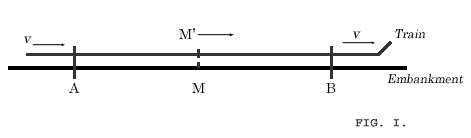reference to the train. Then every event which takes place along the line also takes place at a particular point of the train. Also the definition of simultaneity can be given relative to the train in exactly the same way as with respect to the embankment. As a natural consequence, however, the following question arises: Are two events (e.g. the two strokes of lightning A and B) which are simultaneous with reference to the railway embankment also simultaneous relatively to the train? We shall show directly that the answer must be in the negative. When we say that the lightning strokes A and B are simultaneous with respect to be embankment, we mean: the rays of light emitted at the places A and B, where the lightning occurs, meet each other at the mid-point M of the length A → B of the embankment. But the events A and B also correspond to positions A and B on the train. Let M' be the mid-point of the distance A → B on the travelling train. Just when the flashes (as judged from the embankment) of lightning occur, this point M' naturally coincides with the point M but it moves towards the right in the diagram with the velocity v of the train. If an observer sitting in the position M' in the train did not possess this velocity, then he would remain permanently at M, and the light rays emitted by the flashes of lightning A and B would reach him simultaneously, i.e. they would meet just where he is situated. Now in reality (considered with reference to the railway embankment) he is hastening towards the beam of light coming from B, whilst he is riding on ahead of the beam of light coming from A. Hence the observer will see the beam of light emitted from B earlier than he will see that emitted from A. Observers who take the railway train as their reference-body must therefore come to the conclusion that the lightning flash B took place earlier than the lightning flash A. We thus arrive at the important result: Events which are simultaneous with reference to the embankment are not simultaneous with respect to the train, and vice versa (relativity of simultaneity). Every reference-body (co-ordinate system) has its own particular time; unless we are told the reference-body to which the statement of time refers, there is no meaning in a statement of the time of an event. Now before the advent of the theory of relativity it had always tacitly been assumed in physics that the statement of time had an absolute significance, i.e. that it is independent of the state of motion of the body of reference. But we have just seen that this assumption is incompatible with the most natural definition of simultaneity; if we discard this assumption, then the conflict between the law of the propagation of light in vacuo and the principle of relativity (developed in Section 7) disappears. We were led to that conflict by the considerations of Section 6, which are now no longer tenable. In that section we concluded that the man in the carriage, who traverses the distance w per second relative to the carriage, traverses the same distance also with respect to the embankment in each second of time. But, according to the foregoing considerations, the time required by a particular occurrence with respect to the carriage must not be considered equal to the duration of the same occurrence as judged from the embankment (as reference-body). Hence it cannot be contended that the man in walking travels the distance w relative to the railway line in a time which is equal to one second as judged from the embankment. Moreover, the considerations of Section 6 are based on yet a second assumption, which, in the light of a strict consideration, appears to be arbitrary, although it was always tacitly made even before the introduction of the theory of relativity.

PART II : THE GENERAL THEORY OF RELATIVITY

19. THE GRAVITATIONAL FIELD
 "If we pick up a stone and then let it go, why does it fall to the ground ?" The usual answer to this question is: "Because it is attracted by the earth." Modern physics formulates the answer rather differently for the following reason. As a result of the more careful study of electromagnetic phenomena, we have come to regard action at a distance as a process impossible without the intervention of some intermediary medium. If, for instance, a magnet attracts a piece of iron, we cannot be content to regard this as meaning that the magnet acts directly on the iron through the intermediate empty space, but we are constrained to imagine--after the manner of Faraday--that the magnet always calls into being something physically real in the space around it, that something being what we call a "magnetic field." In its turn this magnetic field operates on the piece of iron, so that the latter strives to move towards the magnet. We shall not discuss here the justification for this incidental conception, which is indeed a somewhat arbitrary one. We shall only mention that with its aid electromagnetic phenomena can be theoretically represented much more satisfactorily than without it, and this applies particularly to the transmission of electromagnetic waves. The effects of gravitation also are regarded in an analogous manner. The action of the earth on the stone takes place indirectly. The earth produces in its surrounding a gravitational field, which acts on the stone and produces its motion of fall. As we know from experience, the intensity of the action on a body dimishes according to a quite definite law, as we proceed farther and farther away from the earth. From our point of view this means : The law governing the properties of the gravitational field in space must be a perfectly definite one, in order correctly to represent the diminution of gravitational action with the distance from operative bodies. It is something like this: The body (e.g. the earth) produces a field in its immediate neighbourhood directly; the intensity and direction of the field at points farther removed from the body are thence determined by the law which governs the properties in space of the gravitational fields themselves. In contrast to electric and magnetic fields, the gravitational field exhibits a most remarkable property, which is of fundamental importance for what follows. Bodies which are moving under the sole influence of a gravitational field receive an acceleration, which does not in the least depend either on the material or on the physical state of the body. For instance, a piece of lead and a piece of wood fall in exactly the same manner in a gravitational field (in vacuo), when they start off from rest or with the same initial velocity. This law, which holds most accurately, can be expressed in a different form in the light of the following consideration. According to Newton's law of motion, we have (Force) = (inertial mass) x (acceleration), where the "inertial mass" is a characteristic constant of the accelerated body. If now gravitation is the cause of the acceleration, we then have (Force) = (gravitational mass) x (intensity of the gravitational field), where the "gravitational mass" is likewise a characteristic constant for the body. From these two relations follows: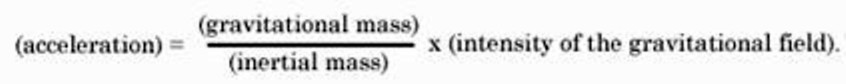If now, as we find from experience, the acceleration is to be independent of the nature and the condition of the body and always the same for a given gravitational field, then the ratio of the gravitational to the inertial mass must likewise be the same for all bodies. By a suitable choice of units we can thus make this ratio equal to unity. We then have the following law: The gravitational mass of a body is equal to its inertial law. It is true that this important law had hitherto been recorded in mechanics, but it had not been interpreted. A satisfactory interpretation can be obtained only if we recognise the following fact : The same quality of a body manifests itself according to circumstances as "inertia" or as "weight" (lit. "heaviness"). In the following section we shall show to what extent this is actually the case, and how this question is connected with the general postulate of relativity.

PART III : CONSIDERATIONS ON THE UNIVERSE AS A WHOLE

SIMPLE DERIVATION OF THE LORENTZ TRANSFORMATION
(SUPPLEMENTARY TO SECTION 11)For the relative orientation of the co-ordinate systems indicated in Fig. 2, the x-axes of both systems permanently coincide. In the present case we can divide the problem into parts by considering first only events which are localised on the x-axis. Any such event is represented with respect to the co-ordinate system K by the abscissa x and the time t, and with respect to the system K' by the abscissa x' and the time t'. We require to find x' and t' when x and t are given.

A light-signal, which is proceeding along the positive axis of x, is transmitted according to the equation

x = ct
or
x - ct = 0 . . . . . (1).

Since the same light-signal has to be transmitted relative to K' with the velocity c, the propagation relative to the system K' will be represented by the analogous formula

x' - ct' = 0 . . . . . (2)

Those space-time points (events) which satisfy (1) must also satisfy (2). Obviously this will be the case when the relation

(x' - ct') = λ(x - ct) . . . (3).

is fulfilled in general, where λ indicates a constant ; for, according to (3), the disappearance of (x - ct) involves the disappearance of (x' - ct').

If we apply quite similar considerations to light rays which are being transmitted along the negative x-axis, we obtain the condition

(x' + ct') = µ(x + ct) . . . (4).

By adding (or subtracting) equations (3) and (4), and introducing for convenience the constants a and b in place of the constants λ and µ, where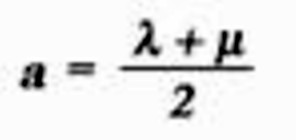and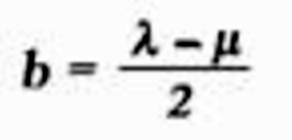we obtain the equations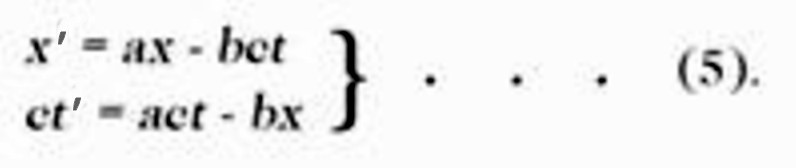We should thus have the solution of our problem, if the constants a and b were known. These result from the following discussion.

For the origin of K' we have permanently x' = 0, and hence according to the first of the equations (5)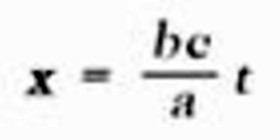If we call v the velocity with which the origin of K' is moving relative to K, we then have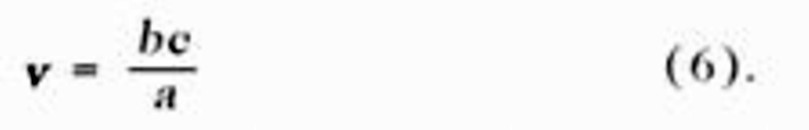The same value v can be obtained from equations (5), if we calculate the velocity of another point of K' relative to K, or the velocity (directed towards the negative x-axis) of a point of K with respect to K'. In short, we can designate v as the relative velocity of the two systems.

Furthermore, the principle of relativity teaches us that, as judged from K, the length of a unit measuring-rod which is at rest with reference to K' must be exactly the same as the length, as judged from K', of a unit measuring-rod which is at rest relative to K. In order to see how the points of the x-axis appear as viewed from K, we only require to take a "snapshot" of K' from K; this means that we have to insert a particular value of t (time of K), e.g. t = 0. For this value of t we then obtain from the first of the equations (5)

x' = ax

Two points of the x'-axis which are separated by the distance Δx' = 1 when measured in the K' system are thus separated in our instantaneous photograph by the distanceBut if the snapshot be taken from K'(t' = 0), and if we eliminate t from the equations (5), taking into account the expression (6), we obtain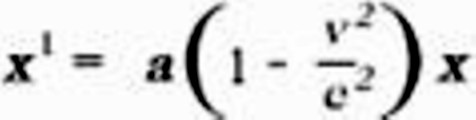From this we conclude that two points on the x-axis separated by the distance 1 (relative to K) will be represented on our snapshot by the distance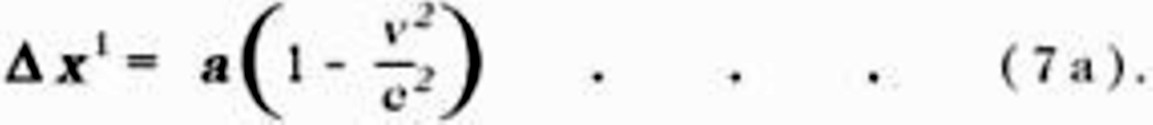But from what has been said, the two snapshots must be identical; hence Δx in (7) must be equal to Δx' in (7a), so that we obtainThe equations (6) and (7b) determine the constants a and b. By inserting the values of these constants in (5), we obtain the first and the fourth of the equations given in Section 11.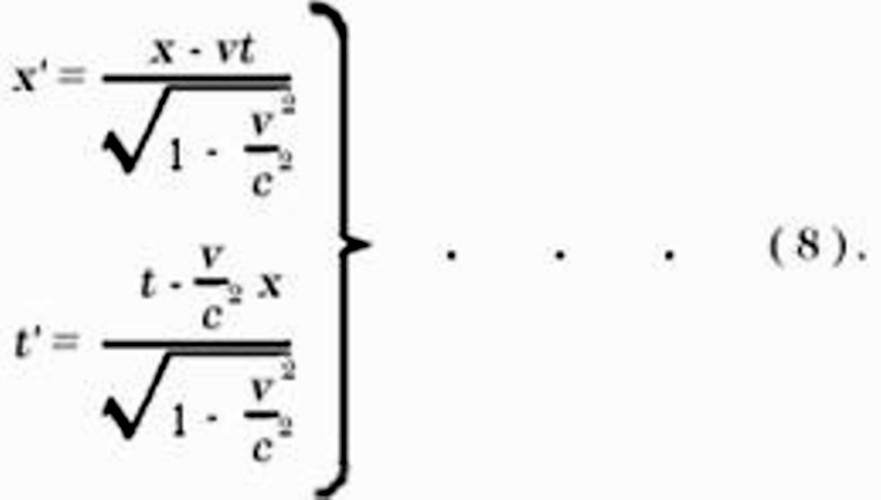Thus we have obtained the Lorentz transformation for events on the x-axis. It satisfies the condition

x'2 - c2t'2 = x2 - c2t2 . . . (8a).

The extension of this result, to include events which take place outside the x-axis, is obtained by retaining equations (8) and supplementing them by the relations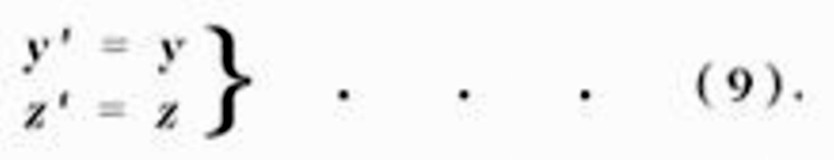In this way we satisfy the postulate of the constancy of the velocity of light in vacuo for rays of light of arbitrary direction, both for the system K and for the system K'. This may be shown in the following manner.

We suppose a light-signal sent out from the origin of K at the time t= 0. It will be propagated according to the equation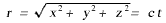or, if we square this equation, according to the equation

x2 + y2 + z2 - c2t2 = 0 . . . (10).

It is required by the law of propagation of light, in conjunction with the postulate of relativity, that the transmission of the signal in question should take place--as judged from K'--in accordance with the corresponding formula

r' = ct'
or,
x'2 + y'2 + z'2 - c2t'2 = 0 . . . . . . (10a).

In order that equation (10a) may be a consequence of equation (10), we must have

x'2 + y'2 + z'2 - c2t'2 = s (x2 + y2 + z2 - c2t2)     (11).

Since equation (8a) must hold for points on the x-axis, we thus have s = 1. It is easily seen that the Lorentz transformation really satisfies equation (11) for s = 1; for (11) is a consequence of (8a) and (9), and hence also of (8) and (9). We have thus derived the Lorentz transformation.

The Lorentz transformation represented by (8) and (9) still requires to be generalised. Obviously it is immaterial whether the axes of K' be chosen so that they are spatially parallel to those of K. It is also not essential that the velocity of translation of K' with respect to K should be in the direction of the x-axis. A simple consideration shows that we are able to construct the Lorentz transformation in this general sense from two kinds of transformations, viz. from Lorentz transformations in the special sense and from purely spatial transformations. which corresponds to the replacement of the rectangular co-ordinate system by a new system with its axes pointing in other directions.

Mathematically, we can characterise the generalised Lorentz transformation thus :

It expresses x', y', x', t', in terms of linear homogeneous functions of x, y, x, t, of such a kind that the relation

x'2 + y'2 + z'2 - c2t'2 = x2 + y2 + z2 - c2t2 (11a).

is satisfied identically. That is to say: If we substitute their expressions in x, y, x, t, in place of x', y', x', t', on the left-hand side, then the left-hand side of (11a) agrees with the right-hand side.

MINKOWSKI'S FOUR-DIMENSIONAL SPACE ("WORLD")
(SUPPLEMENTARY TO SECTION 17)
 We can characterise the Lorentz transformation still more simply if we introduce the imaginary eq. 25 in place of t, as time-variable. If, in accordance with this, we insert          x1 = x          x2 = y          x3 = z          x4 =.eq. 25 and similarly for the accented system K', then the condition which is identically satisfied by the transformation can be expressed thus :          x1'2 + x2'2 + x3'2 + x4'2 = x12 + x22 + x32 + x42 (12). That is, by the afore-mentioned choice of "coordinates," (11a) [see the end of Appendix II] is transformed into this equation. We see from (12) that the imaginary time co-ordinate x4, enters into the condition of transformation in exactly the same way as the space co-ordinates x1, x2, x3. It is due to this fact that, according to the theory of relativity, the "time" x4, enters into natural laws in the same form as the space co ordinates x1, x2, x3. A four-dimensional continuum described by the "co-ordinates" x1, x2, x3, x4, was called "world" by Minkowski, who also termed a point-event a "world-point." From a "happening" in three-dimensional space, physics becomes, as it were, an "existence" in the four-dimensional "world." This four-dimensional "world" bears a close similarity to the three-dimensional "space" of (Euclidean) analytical geometry. If we introduce into the latter a new Cartesian co-ordinate system (x'1, x'2, x'3) with the same origin, then x'1, x'2, x'3, are linear homogeneous functions of x1, x2, x3 which identically satisfy the equation x'12 + x'22 + x'32 = x12 + x22 + x32 The analogy with (12) is a complete one. We can regard Minkowski's "world" in a formal manner as a four-dimensional Euclidean space (with an imaginary time coordinate) ; the Lorentz transformation corresponds to a "rotation" of the co-ordinate system in the four-dimensional "world."

THE EXPERIMENTAL CONFIRMATION OF THE GENERAL THEORY OF RELATIVITY
 From a systematic theoretical point of view, we may imagine the process of evolution of an empirical science to be a continuous process of induction. Theories are evolved and are expressed in short compass as statements of a large number of individual observations in the form of empirical laws, from which the general laws can be ascertained by comparison. Regarded in this way, the development of a science bears some resemblance to the compilation of a classified catalogue. It is, as it were, a purely empirical enterprise. But this point of view by no means embraces the whole of the actual process ; for it slurs over the important part played by intuition and deductive thought in the development of an exact science. As soon as a science has emerged from its initial stages, theoretical advances are no longer achieved merely by a process of arrangement. Guided by empirical data, the investigator rather develops a system of thought which, in general, is built up logically from a small number of fundamental assumptions, the so-called axioms. We call such a system of thought a theory. The theory finds the justification for its existence in the fact that it correlates a large number of single observations, and it is just here that the "truth" of the theory lies. Corresponding to the same complex of empirical data, there may be several theories, which differ from one another to a considerable extent. But as regards the deductions from the theories which are capable of being tested, the agreement between the theories may be so complete that it becomes difficult to find any deductions in which the two theories differ from each other. As an example, a case of general interest is available in the province of biology, in the Darwinian theory of the development of species by selection in the struggle for existence, and in the theory of development which is based on the hypothesis of the hereditary transmission of acquired characters. We have another instance of far-reaching agreement between the deductions from two theories in Newtonian mechanics on the one hand, and the general theory of relativity on the other. This agreement goes so far, that up to the present we have been able to find only a few deductions from the general theory of relativity which are capable of investigation, and to which the physics of pre-relativity days does not also lead, and this despite the profound difference in the fundamental assumptions of the two theories. In what follows, we shall again consider these important deductions, and we shall also discuss the empirical evidence appertaining to them which has hitherto been obtained. (a) Motion of the Perihelion of Mercury According to Newtonian mechanics and Newton's law of gravitation, a planet which is revolving round the sun would describe an ellipse round the latter, or, more correctly, round the common centre of gravity of the sun and the planet. In such a system, the sun, or the common centre of gravity, lies in one of the foci of the orbital ellipse in such a manner that, in the course of a planet-year, the distance sun-planet grows from a minimum to a maximum, and then decreases again to a minimum. If instead of Newton's law we insert a somewhat different law of attraction into the calculation, we find that, according to this new law, the motion would still take place in such a manner that the distance sun-planet exhibits periodic variations; but in this case the angle described by the line joining sun and planet during such a period (from perihelion--closest proximity to the sun--to perihelion) would differ from 360. The line of the orbit would not then be a closed one but in the course of time it would fill up an annular part of the orbital plane, viz. between the circle of least and the circle of greatest distance of the planet from the sun. According also to the general theory of relativity, which differs of course from the theory of Newton, a small variation from the Newton-Kepler motion of a planet in its orbit should take place, and in such away, that the angle described by the radius sun-planet between one perhelion and the next should exceed that corresponding to one complete revolution by an amount given by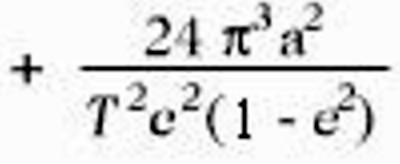(N.B.--One complete revolution corresponds to the angle 2π in the absolute angular measure customary in physics, and the above expression given the amount by which the radius sun-planet exceeds this angle during the interval between one perihelion and the next.) In this expression a represents the major semi-axis of the ellipse, e its eccentricity, c the velocity of light, and T the period of revolution of the planet. Our result may also be stated as follows : According to the general theory of relativity, the major axis of the ellipse rotates round the sun in the same sense as the orbital motion of the planet. Theory requires that this rotation should amount to 43 seconds of arc per century for the planet Mercury, but for the other Planets of our solar system its magnitude should be so small that it would necessarily escape detection.26) In point of fact, astronomers have found that the theory of Newton does not suffice to calculate the observed motion of Mercury with an exactness corresponding to that of the delicacy of observation attainable at the present time. After taking account of all the disturbing influences exerted on Mercury by the remaining planets, it was found (Leverrier: 1859; and Newcomb: 1895) that an unexplained perihelial movement of the orbit of Mercury remained over, the amount of which does not differ sensibly from the above mentioned +43 seconds of arc per century. The uncertainty of the empirical result amounts to a few seconds only. (b) Deflection of Light by a Gravitational Field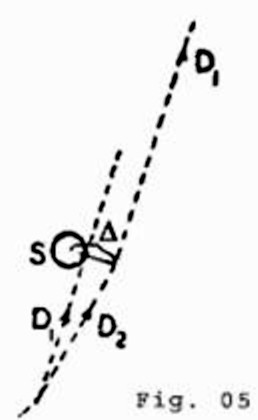In Section 22 it has been already mentioned that according to the general theory of relativity, a ray of light will experience a curvature of its path when passing through a gravitational field, this curvature being similar to that experienced by the path of a body which is projected through a gravitational field. As a result of this theory, we should expect that a ray of light which is passing close to a heavenly body would be deviated towards the latter. For a ray of light which passes the sun at a distance of Δ sun-radii from its centre, the angle of deflection (a) should amount toIt may be added that, according to the theory, half of this deflection is produced by the Newtonian field of attraction of the sun, and the other half by the geometrical modification ("curvature") of space caused by the sun. This result admits of an experimental test by means of the photographic registration of stars during a total eclipse of the sun. The only reason why we must wait for a total eclipse is because at every other time the atmosphere is so strongly illuminated by the light from the sun that the stars situated near the sun's disc are invisible. The predicted effect can be seen clearly from the accompanying diagram. If the sun (S) were not present, a star which is practically infinitely distant would be seen in the direction D1, as observed front the earth. But as a consequence of the deflection of light from the star by the sun, the star will be seen in the direction D2, i.e. at a somewhat greater distance from the centre of the sun than corresponds to its real position. In practice, the question is tested in the following way. The stars in the neighbourhood of the sun are photographed during a solar eclipse. In addition, a second photograph of the same stars is taken when the sun is situated at another position in the sky, i.e. a few months earlier or later. As compared with the standard photograph, the positions of the stars on the eclipse-photograph ought to appear displaced radially outwards (away from the centre of the sun) by an amount corresponding to the angle a. We are indebted to the [British] Royal Society and to the Royal Astronomical Society for the investigation of this important deduction. Undaunted by the [first world] war and by difficulties of both a material and a psychological nature aroused by the war, these societies equipped two expeditions--to Sobral (Brazil), and to the island of Principe (West Africa)--and sent several of Britain's most celebrated astronomers (Eddington, Cottingham, Crommelin, Davidson), in order to obtain photographs of the solar eclipse of 29th May, 1919. The relative discrepancies to be expected between the stellar photographs obtained during the eclipse and the comparison photographs amounted to a few hundredths of a millimetre only. Thus great accuracy was necessary in making the adjustments required for the taking of the photographs, and in their subsequent measurement. The results of the measurements confirmed the theory in a thoroughly satisfactory manner. The rectangular components of the observed and of the calculated deviations of the stars (in seconds of arc) are set forth in the following table of results :(c) Displacement of Spectral Lines Towards the Red In Section 23 it has been shown that in a system K' which is in rotation with regard to a Galileian system K, clocks of identical construction, and which are considered at rest with respect to the rotating reference-body, go at rates which are dependent on the positions of the clocks. We shall now examine this dependence quantitatively. A clock, which is situated at a distance r from the centre of the disc, has a velocity relative to K which is given by          V = wr where w represents the angular velocity of rotation of the disc K' with respect to K. If v0, represents the number of ticks of the clock per unit time ("rate" of the clock) relative to K when the clock is at rest, then the "rate" of the clock (v) when it is moving relative to K with a velocity V, but at rest with respect to the disc, will, in accordance with Section 12, be given byor with sufficient accuracy byThis expression may also be stated in the following form: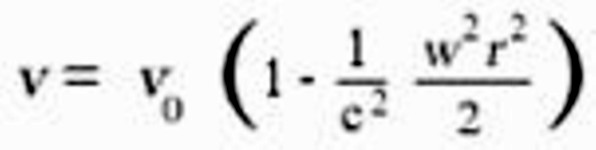If we represent the difference of potential of the centrifugal force between the position of the clock and the centre of the disc by f, i.e. the work, considered negatively, which must be performed on the unit of mass against the centrifugal force in order to transport it from the position of the clock on the rotating disc to the centre of the disc, then we have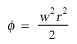From this it follows that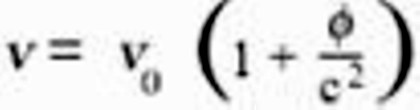In the first place, we see from this expression that two clocks of identical construction will go at different rates when situated at different distances from the centre of the disc. This result is also valid from the standpoint of an observer who is rotating with the disc. Now, as judged from the disc, the latter is in a gravitational field of potential f, hence the result we have obtained will hold quite generally for gravitational fields. Furthermore, we can regard an atom which is emitting spectral lines as a clock, so that the following statement will hold: An atom absorbs or emits light of a frequency which is dependent on the potential of the gravitational field in which it is situated. The frequency of an atom situated on the surface of a heavenly body will be somewhat less than the frequency of an atom of the same element which is situated in free space (or on the surface of a smaller celestial body). Now f = - K (M/r), where K is Newton's constant of gravitation, and M is the mass of the heavenly body. Thus a displacement towards the red ought to take place for spectral lines produced at the surface of stars as compared with the spectral lines of the same element produced at the surface of the earth, the amount of this displacement beingFor the sun, the displacement towards the red predicted by theory amounts to about two millionths of the wave-length. A trustworthy calculation is not possible in the case of the stars, because in general neither the mass M nor the radius r are known. It is an open question whether or not this effect exists, and at the present time (1920) astronomers are working with great zeal towards the solution. Owing to the smallness of the effect in the case of the sun, it is difficult to form an opinion as to its existence. Whereas Grebe and Bachem (Bonn), as a result of their own measurements and those of Evershed and Schwarzschild on the cyanogen bands, have placed the existence of the effect almost beyond doubt, while other investigators, particularly St. John, have been led to the opposite opinion in consequence of their measurements. Mean displacements of lines towards the less refrangible end of the spectrum are certainly revealed by statistical investigations of the fixed stars ; but up to the present the examination of the available data does not allow of any definite decision being arrived at, as to whether or not these displacements are to be referred in reality to the effect of gravitation. The results of observation have been collected together, and discussed in detail from the standpoint of the question which has been engaging our attention here, in a paper by E. Freundlich entitled "Zur Pr?fung der allgemeinen Relativit?ts-Theorie" (Die Naturwissenschaften, 1919, No. 35, p. 520: Julius Springer, Berlin). At all events, a definite decision will be reached during the next few years. If the displacement of spectral lines towards the red by the gravitational potential does not exist, then the general theory of relativity will be untenable. On the other hand, if the cause of the displacement of spectral lines be definitely traced to the gravitational potential, then the study of this displacement will furnish us with important information as to the mass of the heavenly bodies. 27) Notes 26) Especially since the next planet Venus has an orbit that is almost an exact circle, which makes it more difficult to locate the perihelion with precision. 27) The displacement of spectral lines towards the red end of the spectrum was definitely established by Adams in 1924, by observations on the dense companion of Sirius, for which the effect is about thirty times greater than for the Sun. R.W.L. --translator

THE STRUCTURE OF SPACE ACCORDING TO THE GENERAL THEORY OF RELATIVITY
(SUPPLEMENTARY TO SECTION 32)